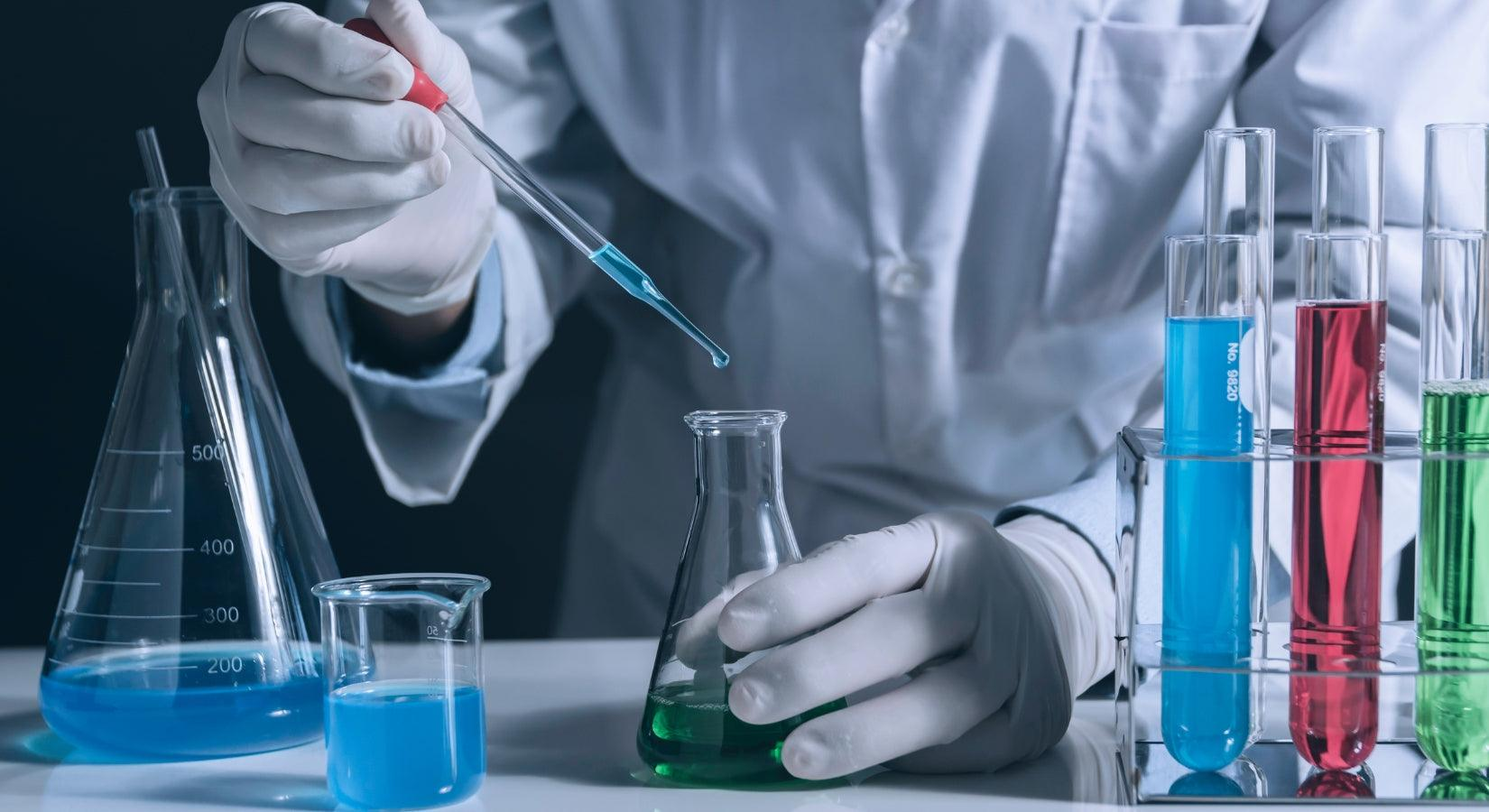HIRANUMA APPLICATION DATA Automatic Titrator Data No. K9 May. 10,2021
 Organic acid Fractional determination of hydrogen peroxide and peracetic acid

## 1. Abstract

Peracetic acid (CH3COOOH) is used as oxidizing agent, bleaching agent, and fungicide. Peracetic acid generates acetic acid and oxygen by its decomposition. It is suggested that the bleaching and antiseptic properties are derived from this generated oxygen. On the other hand, hydrogen peroxide (H2O2) works as both oxidizing and reducing agents depending on the target chemical to be reacted. It is used as fungicide in food industry as well as peracetic acid.

Recently the usage of the mixed solution containing hydrogen peroxide, per acetic acid and acetic acid has been increasing for the sterilization of beverage containers along with the popularization of a plastic bottle as containers. This report introduces an example of fractional determination of hydrogen peroxide and peracetic acid in the mixed solution containing hydrogen peroxide, peracetic acid, and acetic acid.

The fractional titration of hydrogen peroxide and peracetic is performed as follows: first, hydrogen peroxide is titrated with potassium permanganate standard solution (formula(1)) after the sample solution is acidified with sulfuric acid. After the titration, add potassium iodide to generate iodine equivalent to peracetic acid (formula(2)). This generated iodine is titrated with sodium thiosulfate standard solution to determine the peracetic acid (formula(3)).

 2MnO₄⁻ + 5H₂O₂ + 6H⁺ → 2Mn²⁺ + 5O₂(↑) + 8H₂O ・・・(1) CH₃COOOH + 2I⁻ + 2H⁺ → I₂ + H₂O + CH₃COOH ・・・(2) I₂ + 2Na₂S₂O₃ → 2NaI + Na₂S₄O₆ ・・・(3)

## 2. Configuration of instruments and Reagents

 (1) Configuration Main unit : Automatic Titrator COM series Option : One buret Electrode : Platinum electrode　PT-301Reference electrode　RE-201Z* PR-701BZ platinum reference electrode can also be used for measurement instead of above electrodes. (2) Reagents Titrant : 0.02 mol/L Potassium permanganate standard solution0.1 mol/L Sodium thiosulfate standard solution Additive : Diluted sulfuric acid (Sulfuric acid : DI water = 1 : 9 [v/v])1 mol/L Potassium iodide solution

## 3. Measurement procedure

 (1) Take 2 mL of sample into a 100 mL beaker with a volumetric pipette. (2) Add about 50 mL of DI water. (3) Add 2 mL of diluted sulfuric acid. (4) Immerse the electrodes to start titration. The titration with 0.02 mol/L potassium permanganate standard solution for hydrogen peroxide is performed. (5) After the titration of hydrogen peroxide is completed, the waiting time of 120 seconds is automatically counted according to the S-Timer setting. During this waiting time, add 2 mL of 1 mol/L potassium iodide solution using a micropipette. (6) After the S-Timer is finished, the titration with 0.1 mol/L sodium thiosulfate standard solution for peracetic acid are sequentially performed.

## 4. Measurement conditions and results

### Examples of titration conditions

(1) Titration for hydrogen peroxide with potassium permanganate standard solution

 Cndt No. 1 Method Auto Buret No. 1 Amp No. 2 D. Unit mV S-Timer 10 sec C.P. mL 0 mL T Timer 0 sec D.P. mL 0.3 mL End Sens 500 Over mL 0 mL Max Vol. 20 mL Constant No. 1 Size 2 mL Blank 0 mL Molarity 0.02 mol/L Factor 1.003 K 85 L 0 Unit % Formula (D-B)*K*F*M/(S*10) Decimal Places 3 Auto input Param. None Mode No. 5 Pre Int 0 sec Del K 5 Del Sens 0 mV Int Time 3 sec Int Sens 3 mV Brt Speed 2 Pulse 40

(2) Titration for peracetic acid with sodium thiosulfate standard solution

 Cndt No. 3 Method Auto Buret No. 3 Amp No. 2 D. Unit mV S-Timer 120 sec C.P. mL 0 mL T Timer 0 sec D.P. mL 0 mL End Sens 300 Over mL 0.2 mL Max Vol. 20 mL Constant No. 3 Size 2 mL Blank 0 mL Molarity 0.1 mol/L Factor 1.005 K 38.03 L 1 Unit % Formula (D-OA*L)*K*F*M/(S*10) *1 Decimal Places 4 Auto input Param. None Mode No. 5 Pre Int 0 sec Del K 5 Del Sens 0 mV Int Time 3 sec Int Sens 3 mV Brt Speed 2 Pulse 40

*1”OA” and “L” in the formula

The excess potassium permanganate after the titration for hydrogen peroxide reacts with potassium iodide and generates iodine, therefore it must be subtracted. The “OA” in the formula means excess titrated volume of potassium permanganate, the concentration ratio of normality for potassium permanganate standard solution and sodium thiosulfate standard solution is entered to L (Coefficient 2). (“1” is entered on this report because normality of both reagents are 0.1 N.). The excess potassium permanganate is subtracted with this formula.

#### Measurement results

Measurement results of hydrogen peroxide

Meas.
No.
Size
(mL)
Titrant
volume(mL)
Hydrogen peroxide
Concentration (%)
Statistic
calculation
1 2 13.219 1.127 Avg. 1.128 %
2 2 13.217 1.127 SD 0.0023 %
3 2 13.265 1.131 RSD 0.205 %

#### Examples of titration curves#### Measurement results

Measurement results of peracetic acid

Meas.
No.
Size
(mL)
Titrant
volume(mL)
Peracetic acid
Concentration (%)
Statistic
calculation
1 2 2.414 0.4363 Avg. 0.434 %
2 2 2.378 0.4290 SD 0.0041 %
3 2 2.416 0.4359 RSD 0.946 %

#### Examples of titration curves## 5. Note

Hydrogen peroxide and peracetic acid were determined by redox titration. The addition of optional one buret allows to perform fractional titration for hydrogen peroxide and peracetic acid as described in this report. If required, add another optional buret for potassium iodide solution for more automation. However, the potassium iodide solution tends to precipitate, causing clogging of the flow path of buret. Therefore, it is necessary to remove the easily clogged buret tip from the buret for potassium iodide solution, and to clean the flow path with pure water after daily measurement.

Keywords： Hydrogen peroxide, Peracetic acid, Potassium permanganate, Potassium iodide, Sodium thiosulfate, Redox titration

*Some measurement would not be possible depending on optional configuration of system.

Featured Instruments

View all